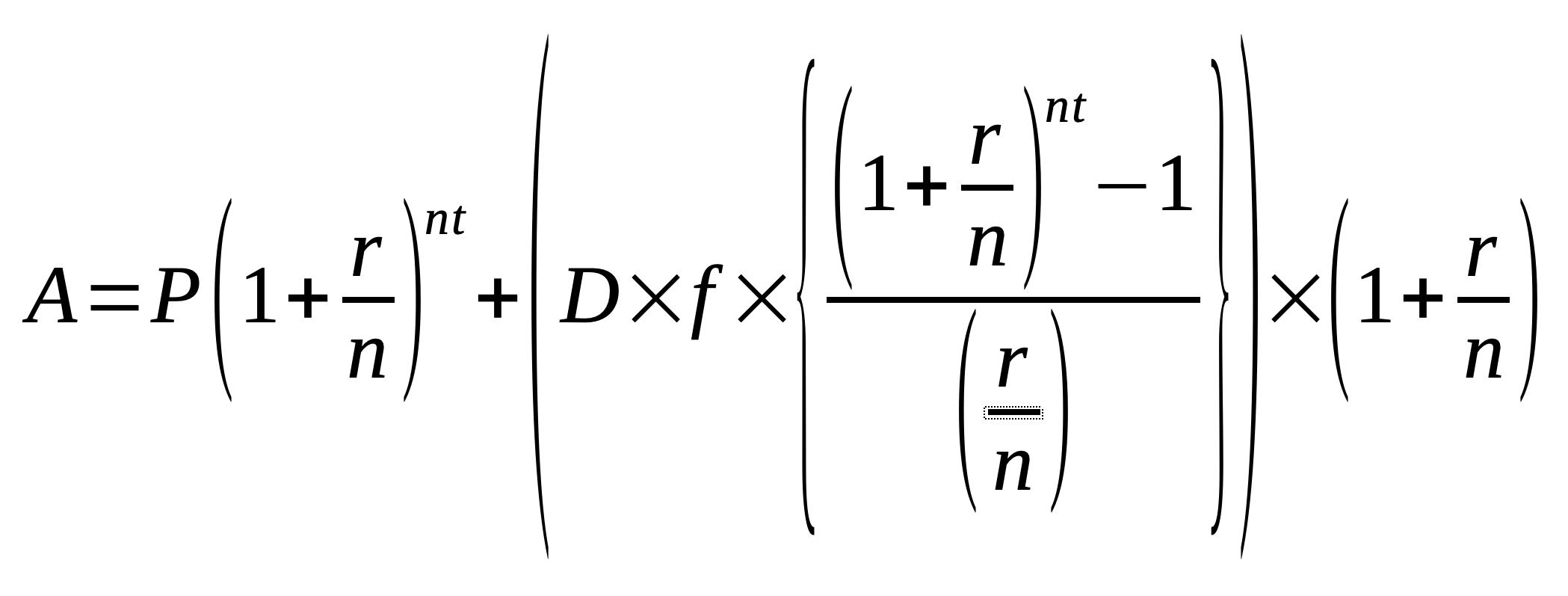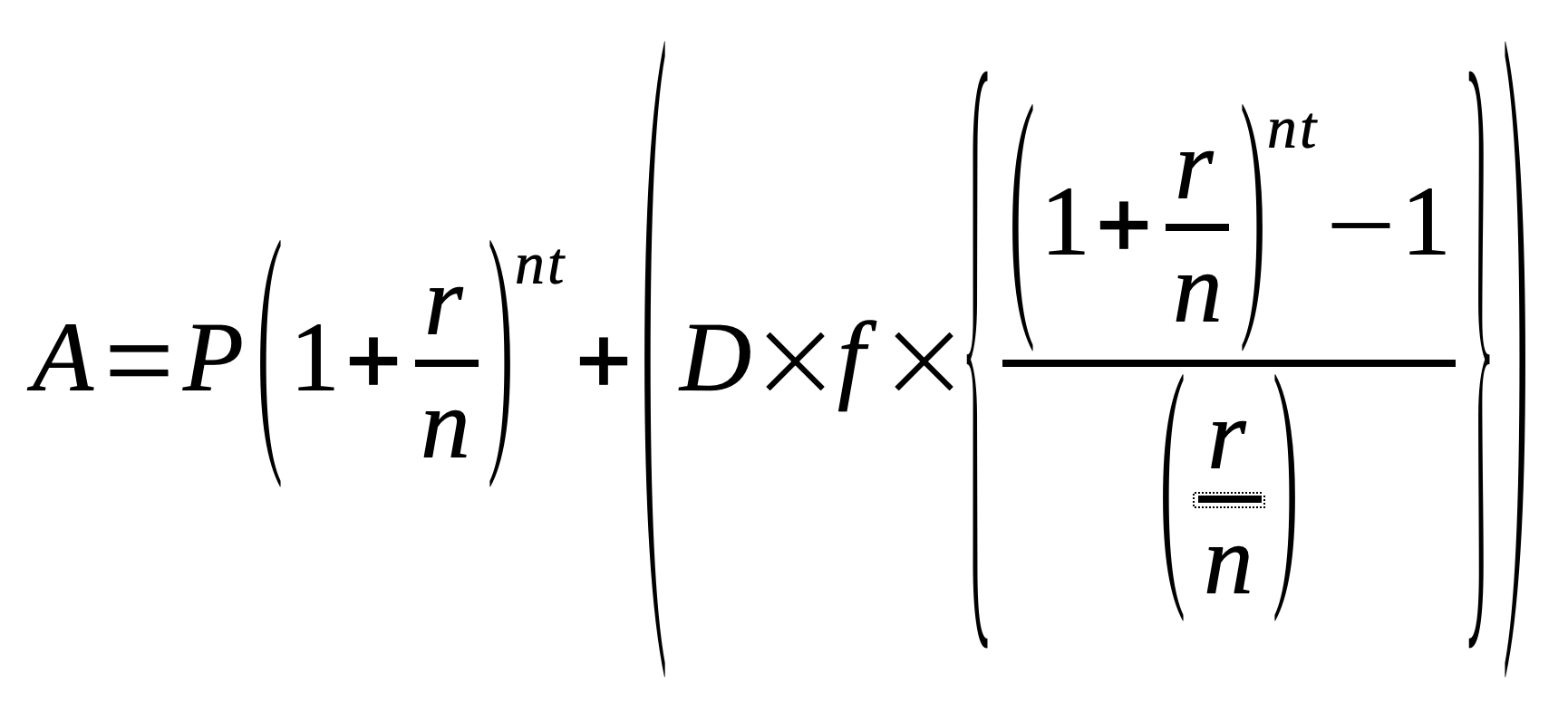# Savings Goal Calculator

Share:

This calculator is used to find when you can reach your savings goal or how much you need to save every month to reach your savings goal.

Enter the required fields and press the Calculate button to get the result.

*
*
\$
*
\$
*
%
*
\$
*
Years
Months
Days
From
ToThis savings goal calculator is used to find:

2. How much you need to save every month to reach your savings goal with the given time duration.

This calculator uses compounding of interest method with options for various compounding periods for interest calculation. It also has an option to add periodic deposits in regular intervals.

### Using Savings Goal Calculator

1. Select the type of calculation you want to perform.
2. Select the Currency of your choice.
3. Enter the Initial Balance amount (principal amount). (NOTE: If you are planning to do only the periodic deposit and not an initial balance, then enter 0 in this field.)
4. Enter the yearly compound Interest Rate in percentage.
5. Select the Compounding Period from the drop down.
6. If you have selected How long it will take to reach my goal, then:
• Enter the Periodic Deposit amount. (NOTE: This is an optional field. So, if you just want to use only the Initial Balance amount then enter 0 in Periodic Deposit field.)
• Select the Frequency of the periodic deposit from the drop down.
7. If you have selected How much to save each month to reach my goal, then:
• Enter the savings Duration either in the form of years, months and days or by specifying the starting and ending date.
8. Finally, press the Calculate Savings button. The final amount, interest accrued and the total deposits will be calculated and the result will be displayed below the button.

## Compound Interest With Initial And Periodic Deposit

There are several types of financial savings. Few of the low-risk or no-risk savings includes bank savings account, High-Yield savings account, CD (Certificate of Deposit), FD (Fixed Deposit), IRA in US, ISA in UK, etc. When you save your money in these accounts, the money will slowly grow with the help of compound interest. Compound Interest is the type of interest calculated on your initial investment amount or principal along with the interest accrued over the previous period of time. i.e., Interest is calculated on the already accrued interest.

### Formula To Find Compound Interest With Just The Initial Deposits

This formula is used when you are doing savings with one time deposit.

A = P (1 + r/n)nt

##### Where:
• A = Final Amount
• P = Principal Amount
• r = Annual Interest Rate (in decimal)
• n = Compounding Period (number of times interest applied per year)
• t = Time (in years)

### Formula To Find Compound Interest With Initial And Periodic Deposit

However, if you like to add more money to your savings by periodically adding a small amount to the initial deposit, then the formula will be different. In fact, there are two formulas based on when you are planning to do the periodic deposit, beginning or end of the period. Let us see the formulas

#### If deposited at the BEGINNING of each period#### If deposited at the END of each period##### Where:
• A = Final Amount
• P = Principal Amount or Initial Balance
• D = Periodic Deposit
• r = Annual Interest Rate (in decimal)
• n = Compounding Period (number of times interest applied per year)
• t = Time (in years)
• f = Number of periodic payments in a compounding period

## Reference

You may also like...